# Bonbons 2

Kilo sweets will cost 260 CZK. The first type has a price per 320 kg, the second type 240 CZK per kg. How many kilos of both kinds of sweets need to prepare a 35 kg mixture ?

Correct result:

x =  8.75 kg
y =  26.25 kg

#### Solution:

320x + 240y = 260(x+y); x+y=35

320•x + 240•y = 260•(x+y)
x+y=35

60x-20y = 0
x+y = 35

x = 35/4 = 8.75
y = 105/4 = 26.25

Our linear equations calculator calculates it.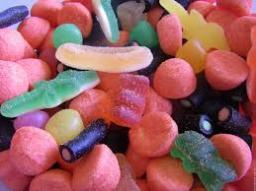We would be pleased if you find an error in the word problem, spelling mistakes, or inaccuracies and send it to us. Thank you!Tips to related online calculators
Do you have a system of equations and looking for calculator system of linear equations?

## Next similar math problems:

• SweetsWe want to prepare 5 kg of sweets for 150 CZK. We will mix cheaper candy: 1 kg for 120 CZK and more expensive candy: 1 kg per 240 CZK. How much of this two types of candy is necessary to prepare this mixture?
• Tea mixture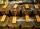Of the two sort of tea at a price of 180 CZK/kg and 240 CZK/kg we make a mixture 12 kg that should be prepared at a price of 200 CZK / kg. How many kilos of each sort of tea will we need to be mixed?
• CandiesThe price for 1 kg of more expensive candies is 125 CZK. The price for 1 kg of cheaper candy is 100 CZK. We mix two different mixtures of candy. A) The first mixture contains 2 kg more expensive and 0.5 kg cheaper candy. Calculate the price for 1 kg of th
• Two teas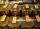A mixture weighing 10 kg was made from two types of tea. The price of tea was 160 CZK/kg and second tea 170 CZK/kg. The price of the mixture is 166 CZK/kg. How many kilograms of each type of tea had to be mixed?
• CandyThe price for 1kg of more expensive candy is 125 CZK. The price for 1kg of cheaper candy is 100 CZK. We make two different blends of candy. And now. The second blend contains 2kg of more expensive candy and several kg of cheaper candy. The price per 1 kg
• BonbonsCreate a mixture of 50 kg of candy on price 700Kč. Candies has prices: 820Kč, 660Kč and 580Kč. Use cross rule.
• Coffee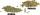Coffee merchant has coffee robusta and arabica species. 1 kg Robusta worth 450 CZK, Arabica 1 kg is 300 CZK more expensive. Calculate how many kilograms of Robusta and Arabica will need to produce 30 kg of the mixture so that the mixture cost is 490 CZK p
• Candies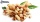In the confectionery, the price for 1 kg of pistachio candies cost CZK 360, and the price for 1 kg of hazelnut candies was CZK 280. Mixing these two types of sweets created a box of chocolates. How many grams of pistachios and how many grams of hazelnut c
• Coffee shop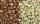To the coffee shop brought 2 types of coffee totally 50 kg. The first type was CZK 220 per kilogram, coffee second type 300 CZK per 1 kg. For all the coffee trader earned CZK 12,000. How many kilograms of coffee of first type and how many kilograms of cof
• ConcertOn a Concert were sold 150 tickets for CZK 360, 235 tickets for 240 CZK and 412 for 180 CZK. How much was the total revenues for tickets?
• Mixture of nutsThe mixture of nuts should be prepared from almonds, peanuts and cashew nuts ratio 1: 2: 3 (respectively). The price of almonds is 150 CZK/kg, the price of peanuts is 140 CZK/kg and the price of cashew nuts is 180 CZK/kg. The price of the mixture is deter
• Luggage and air travelTwo friends traveling by plane had a total of 35 kg of luggage. They paid one 72 CZK and second 108 CZK for being overweight. If only one paid for all the bags, it would cost 300 CZK. What weight of baggage did each of them have, how many kilograms of lug
• Bananas and mango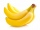5 kg of bananas and three kilograms of mango cost 146 CZK, two kilograms of bananas and 5 kilograms of mango cost 142 CZK. How much is a kilogram of bananas, and how many mangoes?
• Toys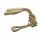The toy shop sold the same number of balls for 62 CZK and skipping ropes for 34 CZK. They income was 576 CZK. How many sold balls and how many skipping ropes?
• Purchase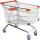Mother bought 5 boxes of milk and 7 kg of potatoes and paid a total CZK 147. Aunt bought 7 boxes of milk and 3 kg of potatoes and paid 131 CZK. What is the price of one carton of milk and 1 kg of potatoes? How CZK together would have saved if bought at th
• Tea blendTea blends are maked from two kinds of tea. In standard tea mixture are two teas in the ratio 1:3 and 40 g costs 42 CZK. In the premium tea mixture are weighing two teas in the ratio 1:1 and 50 grams costs 60 CZK. How much cost 10 grams of more expensive
• Dried fruit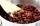The manufacturer produces a mixture of dried fruit. He purchased: 10kg pineapple for 200 Kc/kg 2kg papaya for 180 kc/kg 1kg of banana for 400 Kc/kg How many kgs of raisin for 80 Kc/kg must be put into the mix by the manufacturer so that the production pri